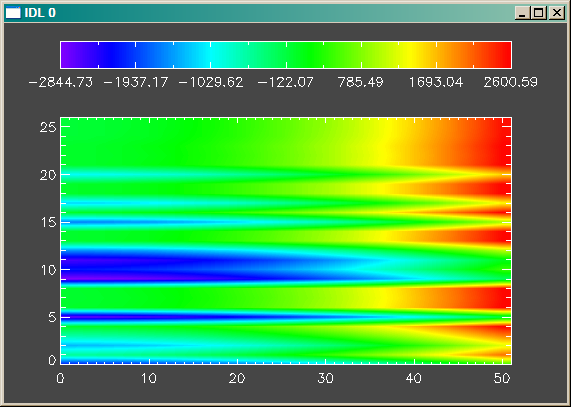Display a Function of Two Variables

QUESTION: I have two vectors, X and Y, and I would like to display a function of these two vectors, f(X,Y), as an image with a colorbar representing the values of the function. Can you help me do this?ANSWER: The only trick here is to know how to construct the appropriate 2D arrays from your X and Y vectors. Dimensional juggling skills are the essential arrows you need in your quill.

First, let's construct a couple of vectors to illustrate. In this particular case, the X vector will have 51 elements and the Y vector will have 26 elements.

x = Findgen(51)    y = RandomU(-3L, 26) * 10

Now we use our dimensional juggling skills to create 2D arrays of the X and Y vectors.

xarray = Rebin(x, 51, 26)    yarray = Rebin(Reform(y, 1, 26), 51, 26)

Next, we create the function of these two variables.

f = xarray^2 - 3*yarray^3 + 2*(xarray + yarray)

Finally, we display the function of these two variables as an image, along with a color bar representing the value of the function. We use the Coyote Library routines TVImage and FSC_Colorbar in this step.

TVImage, BytScl(f, TOP=254), Position=[0.1, 0.1, 0.9, 0.75], \$
AXES=1, /ERASE, BACKGROUND='charcoal'
FSC_Colorbar, RANGE=[Min(f), Max(f)], FORMAT='(F0.2)', NCOLORS=254

You can see the results in the figure below.A function of two variables displayed as an image.

Naturally, you can also display this function as a surface or contour plot if you would prefer.